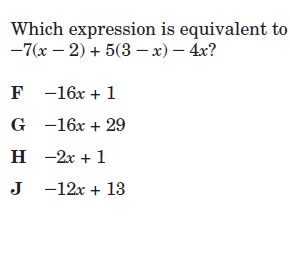GeoB enrichment 2
When finished email and let me know you have completed this
Exponents *
1 point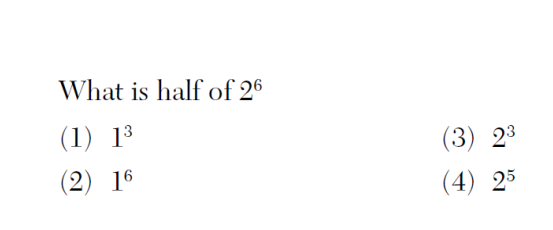Which is parallel to the line y = -4 + 7 *
1 point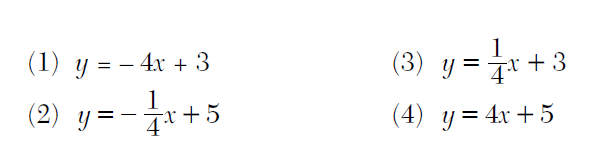1 point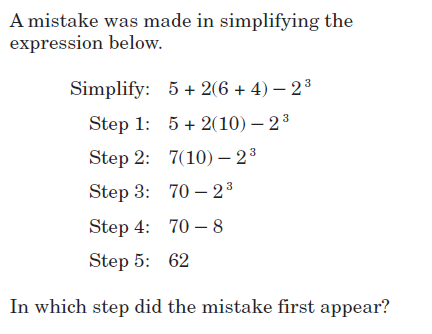*
1 point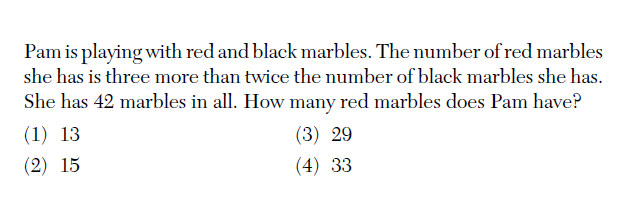Rates *
1 point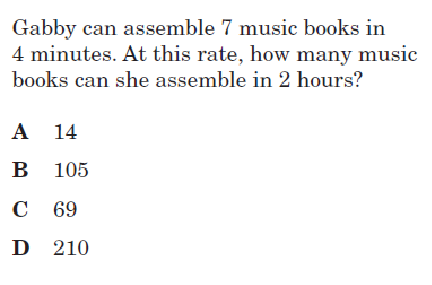slope *
1 point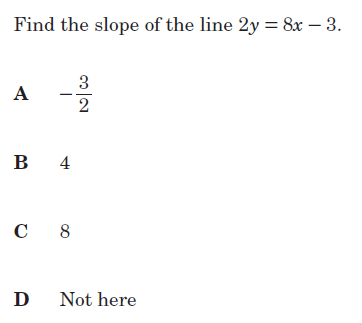Rates *
1 point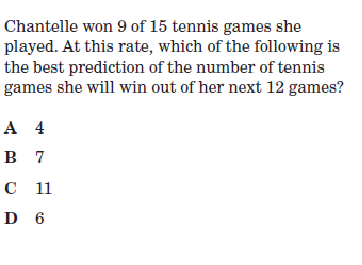Name *
What is the slope of the line that passes through the points (–6,1)and (4,–4)? *
1 point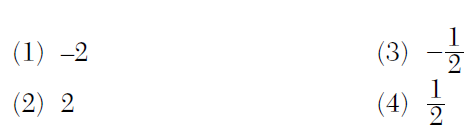functions *
1 point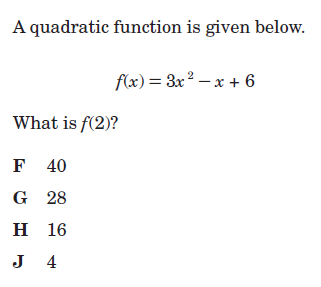Rates *
1 point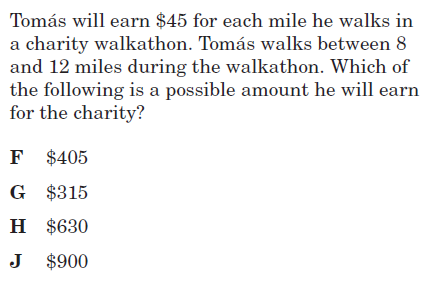Rates *
1 point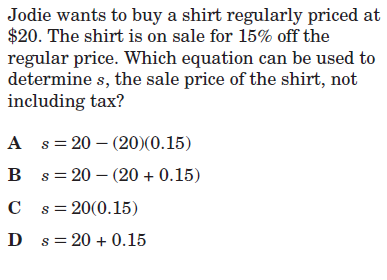polynomials *
1 point